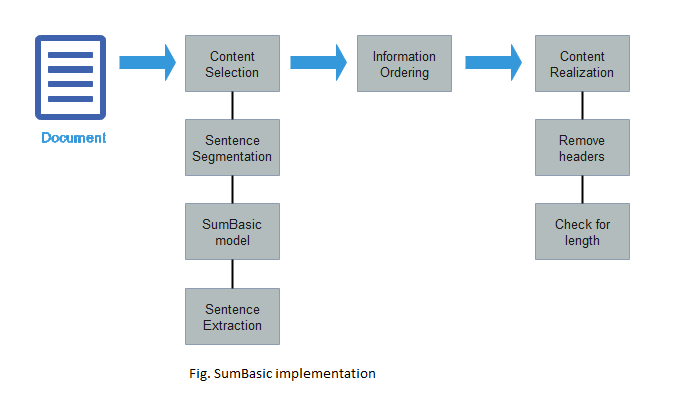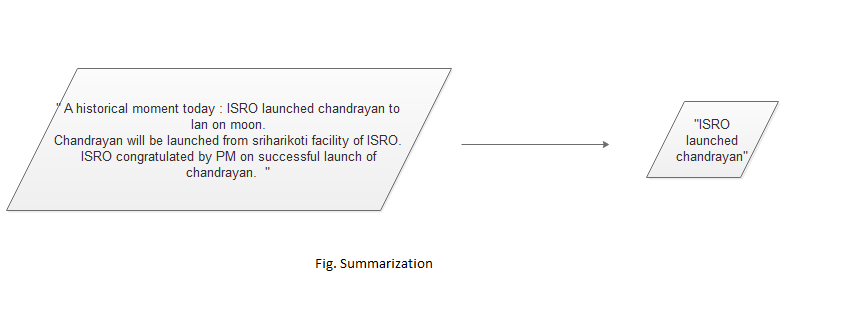×

Search anything:

# SumBasic algorithm for text summarization

#### Machine Learning (ML) Natural Language Processing (NLP)Indian Technical Authorship Contest starts on 1st July 2023. Stay tuned.

Reading time: 30 minutes | Coding time: 10 minutes

SumBasic is an algorithm to generate multi-document text summaries. Basic idea is to utilize frequently occuring words in a document than the less frequent words so as to generate a summary that is more likely in human abstracts.

It generates n length summaries, where n is user specified number of sentences.

SumBasic has the following advantages :

1. Used to easily understand the purpose of a document.
2. Provides greater convinience and flexibility to reader.
3. Generates shorter and concise form from multiple documents.The above figure shows the working of SumBasic on a document.

## Alogorithm

SumBasic follows the following algorithm :

1. It calculates the probability distribution over words w appearing in the input P(wi) for every i

p(wi)=n/N

Where,
n = number of times the word appeared in the input.
N = total number of content word tokens in input.

1. For sentence Sj in input, assign a weight equal to the average probability of the words in the sentence.

Sj=p(wi)/|wi|

1. Pick the best scoring sentence with highest probability word.

2. For each word wi in the sentence chosen at step 3, update their probability.

*

pnew(wi)=pold(wi)pold(wi)

1. If a desired summary length is not generated then repeat the step 2.

The step 2 and 3 exhibits the desired properties of summarizer whereas step 3 ensures that the highest probability word is included in the summary every time a sentence is picked.
Step 4 has various uses; updating probabilities on the basis of preceding sentences so that low probability words can also participate and deals with the redundacies.

In simple words, SumBasic first computes the probability of each content-word (i.e., verbs, nouns, adjectives and numbers) by simply counting its frequency in the document set. Each sentence is scored as the average of the probabilities of the words in it. The summary is then generated through a simple greedy search algorithm: it iteratively selects the sentence with the highest-scoring content-word, breaking ties by using the average score of the sentences. This continues until the maximum summary length has been reached. In order not to select the same or similar sentence multiple times, SumBasic updates probabilities of the words in the selected sentence by squaring them, modeling the likelihood of a word occurring twice in a summary.

### Complexity analysis

Worst case : O(2 * n + n * (n^3 + n * log(n) + n^2)
given by : O(step 1 complexity + n*(step 2, 3, and 4 complexity))

# Implementation

SumBasic can be implemented using sumy or nltk library in python

Sumy installation command :

``````pip install sumy
``````

nltk installation command:

``````pip install nltk
``````

sumy is used to extract summary from html pages or plain texts.

The data is processed through various steps to undergo the procedure of summarization :

1. Tokenization - A sentence is split into words as tokens which are then processed to find the distinct words.
2. Stemming - Stemming is used filter out the parent word from any word . For ex : having will be converted into have by stemming out "ing".
3. Lemmatization - Lemmatization is the process of converting a word to its base form. The difference between stemming and lemmatization is, lemmatization considers the context and converts the word to its meaningful base form, whereas stemming just removes the last few characters, often leading to incorrect meanings and spelling errors.

There are three ways to implement the algorithm, namely:

1. orig: The original version, including the non-redundancy update of the word scores.
2. simplified: A simplified version of the system that holds the word scores constant and does not incorporate the non-redundancy update. It produces better results than orig version in terms of simplification.
3. leading: Takes the leading sentences of one of the articles, up until the word length limit is reached. It is the most concise technique.

NOTE- The code implemented below does not use sumy.

## Sample Code

``````import nltk, sys, glob

sys.setdefaultencoding('utf8')

lemmatize = True
rm_stopwords = True
num_sentences = 10
stopwords = nltk.corpus.stopwords.words('english')
lemmatizer = nltk.stem.WordNetLemmatizer()

#Breaking a sentence into tokens

def clean_sentence(tokens):
tokens = [t.lower() for t in tokens]
if lemmatize: tokens = [lemmatizer.lemmatize(t) for t in tokens]
if rm_stopwords: tokens = [t for t in tokens if t not in stopwords]

def get_probabilities(cluster, lemmatize, rm_stopwords):

# Store word probabilities for this cluster

word_ps = {}

# Keep track of the number of tokens to calculate probabilities later

token_count = 0.0

# Gather counts for all words in all documents

for path in cluster:
with open(path) as f:
token_count += len(tokens)
for token in tokens:
if token not in word_ps:
word_ps[token] = 1.0
else:
word_ps[token] += 1.0

# Divide word counts by the number of tokens across all files

for word_p in word_ps:
word_ps[word_p] = word_ps[word_p]/float(token_count)
return word_ps

def get_sentences(cluster):
sentences = []
for path in cluster:
with open(path) as f:
return sentences

def clean_sentence(tokens):
tokens = [t.lower() for t in tokens]
if lemmatize: tokens = [lemmatizer.lemmatize(t) for t in tokens]
if rm_stopwords: tokens = [t for t in tokens if t not in stopwords]

def score_sentence(sentence, word_ps):
score = 0.0
num_tokens = 0.0
sentence = nltk.word_tokenize(sentence)
tokens = clean_sentence(sentence)
for token in tokens:
if token in word_ps:
score += word_ps[token]
num_tokens += 1.0
return float(score)/float(num_tokens)

def max_sentence(sentences, word_ps, simplified):
max_sentence = None
max_score = None
for sentence in sentences:
score = score_sentence(sentence, word_ps)
if score > max_score or max_score == None:
max_sentence = sentence
max_score = score
if not simplified: update_ps(max_sentence, word_ps)
return max_sentence

# Updating the sentences , step 4 of algo

def update_ps(max_sentence, word_ps):
sentence = nltk.word_tokenize(max_sentence)
sentence = clean_sentence(sentence)
for word in sentence:
word_ps[word] = word_ps[word]**2
return True

def orig(cluster):
cluster = glob.glob(cluster)
word_ps = get_probabilities(cluster, lemmatize, rm_stopwords)
sentences = get_sentences(cluster)
summary = []
for i in range(num_sentences):
summary.append(max_sentence(sentences, word_ps, False))
return " ".join(summary)

def simplified(cluster):
cluster = glob.glob(cluster)
word_ps = get_probabilities(cluster, lemmatize, rm_stopwords)
sentences = get_sentences(cluster)
summary = []
for i in range(num_sentences):
summary.append(max_sentence(sentences, word_ps, True))
return " ".join(summary)

cluster = glob.glob(cluster)
sentences = get_sentences(cluster)
summary = []
for i in range(num_sentences):
summary.append(sentences[i])
return " ".join(summary)

def main():
method = sys.argv
cluster = sys.argv
summary = eval(method + "('" + cluster + "')")
print summary

if __name__ == '__main__':
main()
``````

Let following picture represents how the above code will be executed :## Applications

Data summarization has huge boudaries of its application from extracting relevant informations to dealing with redundancies. Some of the major applications are as follows :

1. Information retrieval by Google , Yahoo, Bing and so on. Whenever a query is encountered , thousands of pages appear . It becomes difficult to extract the relevant and significant information from them . Summarization is done in order to prevent this .
3. Summary of source of text in shorter version is provided to the user that retains all the main and relevant features of the content.
4. Easily understandable

### Other Summarization techniques can be as follows :

1. LexRank
2. TextRank
3. Latent Semantic Analysis(LSA) and so on.

## Questions

1. Are NLP and SumBasic different?

SumBasic in a technique to implement NLP. It is advantageous as it investigate how much the frequency of words in the cluster of input documents influences their selection in the summary.

1. How are tf-idf and SumBasic different?

Tf-idf stands for term "frequency–inverse document frequency" in which it considers both the frequency of the term in a document and inverse document frequency that is pages of document in which the required terms exist , whereas in SumBasic uses maximum frequent element and so on in decreasing order to determine the context of document or text. Tf-idf is used more frequently used in chatbots and where the machine has to understand the meaning and communicate with the user, whereas summarization is used to provide user with concise information.

1. Lucy Vanderwend, Hisami Suzuki et al, Beyond SumBasic: Task-Focused Summarization with Sentence Simplification and Lexical Expansion
2. Chintan Shah & Anjali Jivani, (2016). LITERATURE STUDY ON MULTI-DOCUMENT TEXT SUMMARIZATION TECHNIQUES. In SmartCom., September-2016. Jaipur: Springer.
3. A SURVEY OF TEXT SUMMARIZATION TECHNIQUES :Ani Nenkova,University of Pennsylvania;Kathleen McKeown,Columbia University.#### Ashutosh Vashisht

B.Tech (IT) Student at Amity School of Engineering and Technology